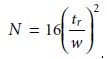Home | | Modern Analytical Chemistry | Column Efficiency - Theory of Column Chromatography

# Column Efficiency - Theory of Column Chromatography

At the beginning of a chromatographic separation the solute occupies a narrow band of finite width.

Column Efficiency

At the beginning of a chromatographic separation the solute occupies a narrow band of finite width. As the solute passes through the column, the width of its band continually increases in a process called band broadening. Column efficiency pro- vides a quantitative measure of the extent of band broadening.

In their original theoretical model of chromatography, Martin and Synge2 treated the chromatographic column as though it consists of discrete sections at which partitioning of the solute between the stationary and mobile phases occurs. They called each section a theoretical plate and defined column efficiency in terms of the number of theoretical plates, N, or the height of a theoretical plate, H; where12.12

A columnŌĆÖs efficiency improves with an increase in the number of theoretical plates or a decrease in the height of a theoretical plate.

Assuming a Gaussian profile, the extent of band broadening is measured by the variance or standard deviation of a chromatographic peak. The height of a theoreti- cal plate is defined as the variance per unit length of the column12.13

where the variance, Žā2, has units of distance squared. Because retention time and peak width are usually measured in seconds or minutes, it is more convenient to ex- press the standard deviation in units of time, Žä, by dividing  by the mobile phaseŌĆÖs average linear velocity.12.14

When a chromatographic peak has a Gaussian shape, its width at the baseline, w, is four times its standard deviation, Žä.

w = 4Žä            ŌĆ”ŌĆ”ŌĆ”ŌĆ”ŌĆ”ŌĆ”ŌĆ”12.15

Combining equations 12.13 through 12.15 gives the height of a theoretical plate in terms of the easily measured chromatographic parameters tr and w.12.16

The number of theoretical plates in a chromatographic column is obtained by com- bining equations 12.12 and 12.16.12.17

Alternatively, the number of theoretical plates can be approximated aswhere w1/2 is the width of the chromatographic peak at half its height.It is important to remember that a theoretical plate is an artificial construct and that no such plates exist in a chromatographic column. In fact, the number of theo- retical plates depends on both the properties of the column and the solute. As a re- sult, the number of theoretical plates for a column is not fixed and may vary from solute to solute.

Study Material, Lecturing Notes, Assignment, Reference, Wiki description explanation, brief detail
Modern Analytical Chemistry: Chromatographic and Electrophoretic Methods : Column Efficiency - Theory of Column Chromatography |